# Spacecraft Attitude Determination Using Global Positioning Satellite Signals

 The second method is the integer search method. Essentially, all possible orientations of the satellite for the given phase differences are worked through to determine which orientation is the best match. This method is computationally demanding and time-consuming for large spacecraft in which several orientations are possible for a given phase difference (Global Positioning System: Theory and Applications Volume II). A third method for integer resolution is to use several baselines of various sizes. The integer phase difference for small baselines is easily determined and this knowledge allows for the resolution of larger baselines until all baselines have been resolved. This method is unattractive as it requires additional hardware, more complex software, and is computationally inefficient (Global Positioning System: Theory and Applications Volume II). A fourth method for integer resolution is the use of dual-frequency receivers. By measuring two phase differences between the two receivers, the integer phase difference for both frequencies can be determined. Although computationally efficient, it requires more complicated and expensive hardware (Global Positioning System: Theory and Applications Volume II). Once the integer phase difference is accounted for, the error between the measured phase difference and that calculated from the transformation matrix must be minimized. The GPS loss function is defined as: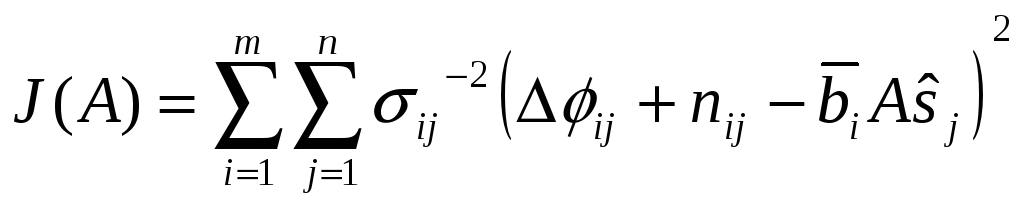(3) In which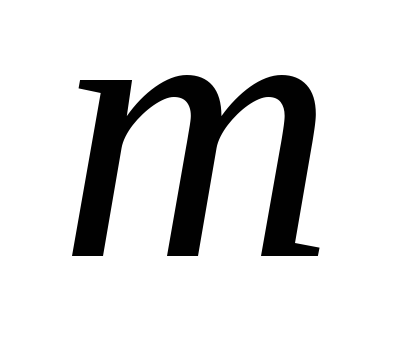is the total number of baselines,is the total number of sightlines, andis the standard deviation of the GPS signal in wavelengths (Efficient and Optimal Attitude Determination Using Recursive Global Positioning System Signal Operations). There are several methods that have been used to minimize the loss function. Perhaps the most obvious is the linear least squares method. Although simple in concept, it uses motion-based integer resolution incorporated into the algorithm. It also possesses the problem that it diverges for poor initial guesses. The altered loss function is shown below: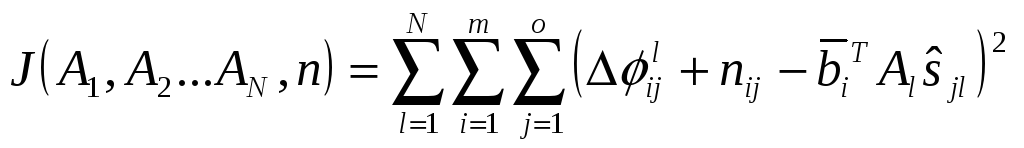(4) For which l is the epoch and Al is the attitude matrix at that epoch (Global Positioning System: Theory and Applications Volume II). A second method that has been tried and tested is the use of a predictive filter in an algorithm called ALLEGRO (Attitude-Lean-Loping-Estimator using GPS Recursive Operations). Although possessing the advantages that it is non-iterative and always converges with a minimum number of baselines and sightlines (either two baselines and three sightlines or three baselines and two sightlines), it does not incorporate an integer resolution method, but assumes the integer portion is compensated for. The loss function for this algorithm is: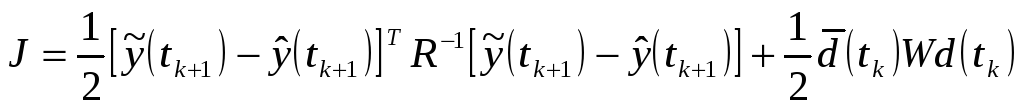(5) For which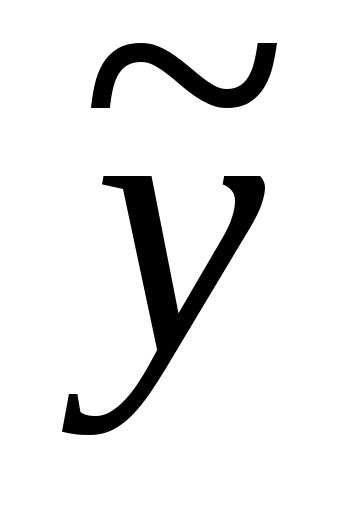is the measurement vector at a given time,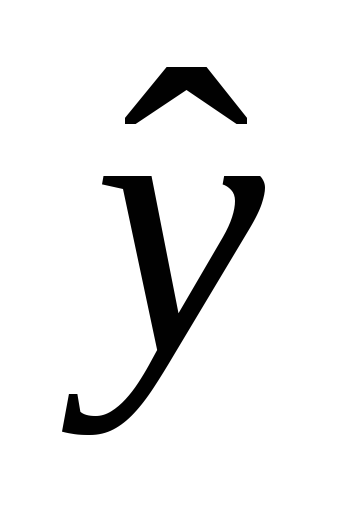is the estimate,is a weighting matrix,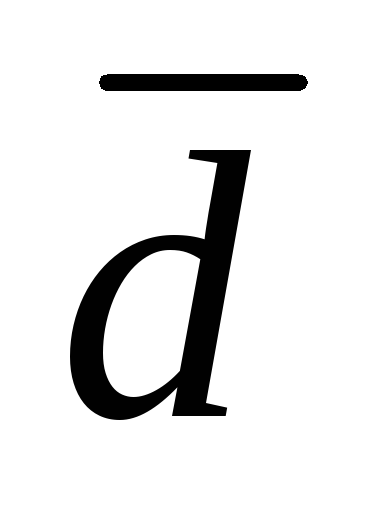is an error matrix, andis a second weighting matrix (Efficient and Optimal Attitude Determination Using Recursive Global Positioning System Signal Operations). This paper will explore a method of non-motion-based integer resolution and an algorithm for determining a spacecraft attitude using a minimum number of receivers. Gauss-Newton and Gauss-Newton-Levenberg-Marquardt iterations are then used for attitude determination. Download 1.81 Mb.Share with your friends: